Get instant live expert help with Excel or Google Sheets“My Excelchat expert helped me in less than 20 minutes, saving me what would have been 5 hours of work!”

#### Post your problem and you’ll get Expert help in seconds.

Your message must be at least 40 characters
Our professional Expert are available now. Your privacy is guaranteed.

# Learn How to Count Unique Values in a Column

In what situations would you need to count distinct values in a column? While working on data, many times we come across a case where we have a data set that contains duplicate values, and we want to know that how many values are distinct there.

In small data set it is easy to identify and count distinct values manually out of duplicates, but in large data set it is hard to do that. In Excel, there are several ways to count distinct values in a column. In this article, we will discuss the following widely used ways for this purpose.

## Count unique values in a column in Excel

### Find all distinct values in a column using the Advanced Filter

Using the Advanced Filter dialog box feature, you can easily extract distinct values from a column and paste them in a separate location in the worksheet. Later you can count a new list of distinct values using ROWS or COUNTA function.

Suppose we have a list of City names in a column which contains duplicate values, and we want to get the list of distinct values using Advanced Filter. To do that, please perform the following steps.

• Select the data range in a column and make sure to include column heading in the selection, if the column has heading name, such as “City.”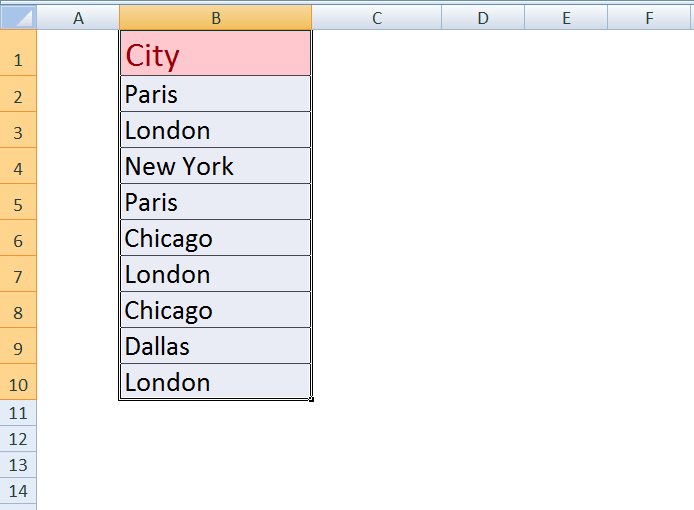• On the Data tab, in the Sort & Filter group, click Advanced. The Advanced Filter dialog box appears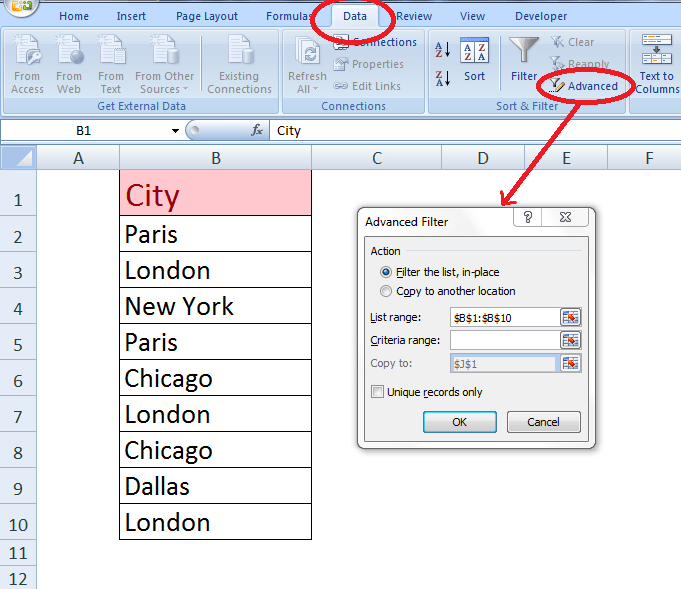• Then Click Copy to another location option
• In the Copy to box, enter a cell reference where you want to paste new list. Alternatively, click Collapse Dialog, select a cell on the worksheet, and then press Expand Dialog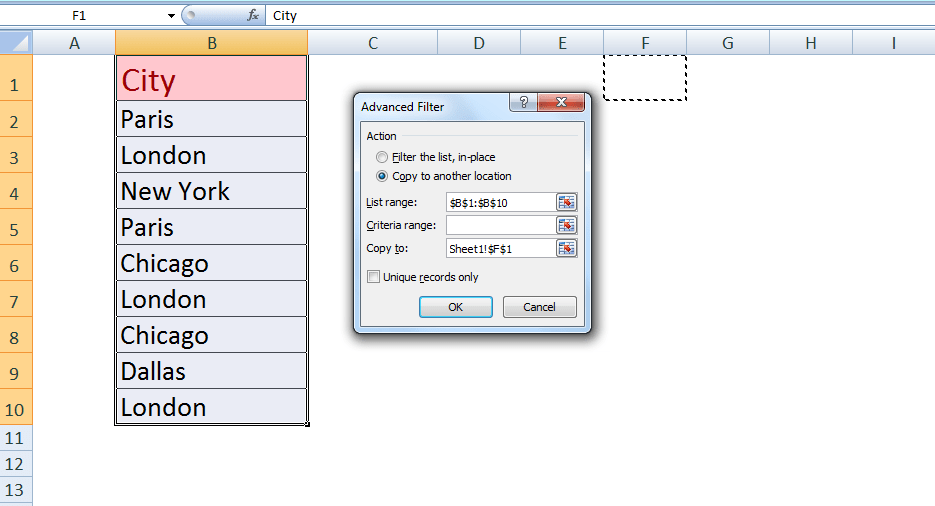• ➢Select Unique records only check box, and press OK button
A new list of distinct values from a selected range of cells in a column is copied to a new location, which you specified in Copy to the dialog box.
• ➢Using ROWS or COUNTA function, you can easily count the values of a newly generated list of distinct values (excluding column header in selection), such as `=ROWS(F2:F6)`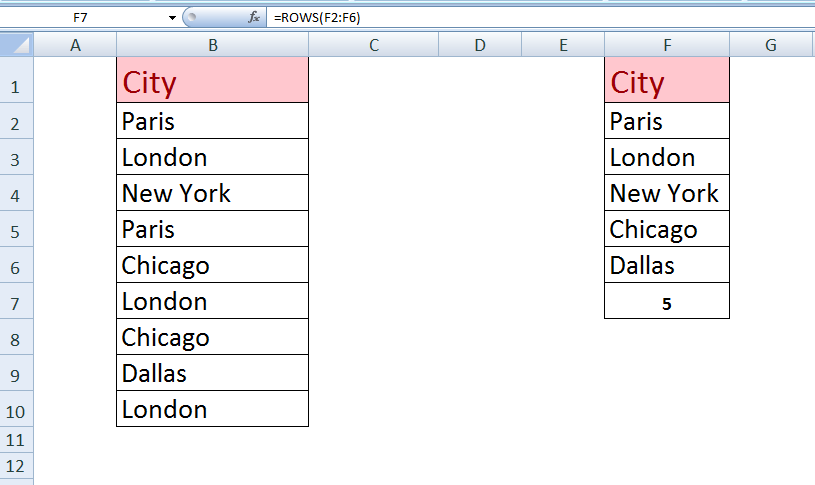### Count number of distinct values in a column using a Formula

Using a formula you can directly count the number of distinct values in a column. This formula must be used as an array formula, and it is a combination of multiple functions of Excel to do so.

This formula has two versions. Each version is used with some variation for numeric values only or both text and numeric values in a list.

The formula is a combination of SUM, IF, FREQUENCY, MATCH functions. To convert this formula to an array formula, you must press CRT+SHIFT+ENTER instead of just pressing ENTER after inserting a formula in an active cell.

• FREQUENCY function calculates how often values occur within a range of values or cells, and then returns a vertical array of numbers. That’s why this formula is used as an array formula. This function ignores text or zero values.
• MATCH function searches for a specified value in a range of values and then returns its relative position in a selected range of values.
• IF function returns a value if condition or argument specified in the logical test is TRUE. Here a value of 1 is assigned to each TRUE condition in IF function.
• SUM function adds the numbers that you specify in the argument.

1st Version
This version is used to count the number of distinct numeric values only in a cells range, such as B2:B10. It does not count text values or blank cells in the range.
`=SUM(IF(FREQUENCY(B2:B10,B2:B10)>0,1))`

As FREQUENCY function ignores Text or zero values, so only numeric values can be counted in this version of the formula. By assigning a value of 1 to each TRUE condition, an array of 1s and FALSE values is generated, and SUM function adds all 1s values to count distinct values in cells.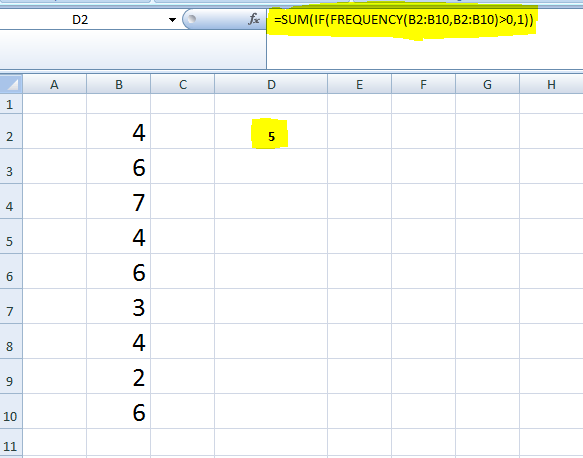By pressing function key F9, we can see the result of this formula in the formula bar.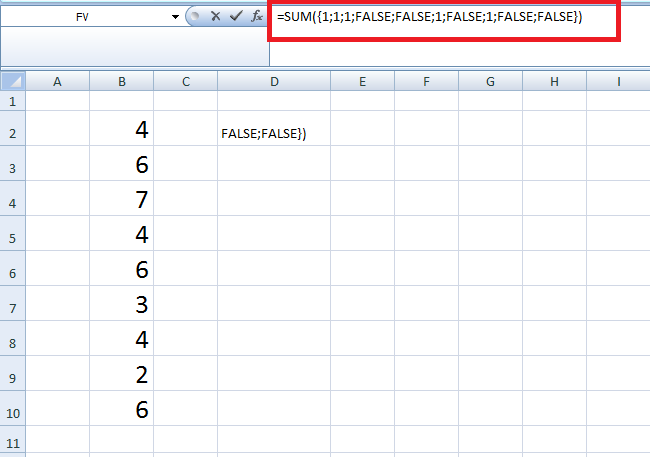2st Version
This version can be used for both numeric and text values in a column. This counts the number of distinct numbers and text values in a column or cells, like B2:B10, but cells must not contain blank cells.
`=SUM(IF(FREQUENCY(MATCH(B2:B10,B2:B10,0),MATCH(B2:B10,B2:B10,0))>0,1))`

The MATCH function returns the relative position of both text and numeric values, so this function counts the number of distinct text and numeric values both. Suppose we have a list of text value in cells that have duplicate values, then using this formula we can count distinct text values only as shown below.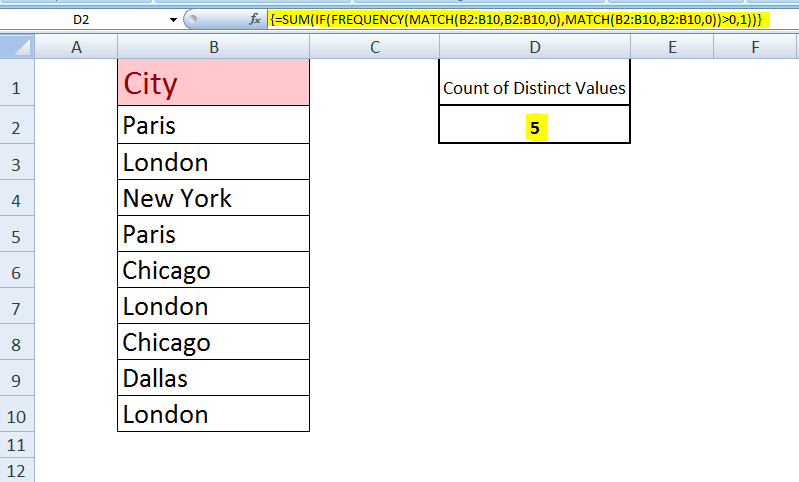### Find all distinct values and frequency using Pivot table

This functionality is available in the latest versions of Excel, Excel 2013 and Excel 2016 only. For creating a Pivot Table with a distinct count for the desired column follow these steps.

• Select the data range, go to Insert TabTables group, and click the PivotTable button.
• Create Pivot Table dialog pops up. Choose whether to place your pivot table in a new or existing worksheet and make sure to select Add this data to the Data Model checkbox.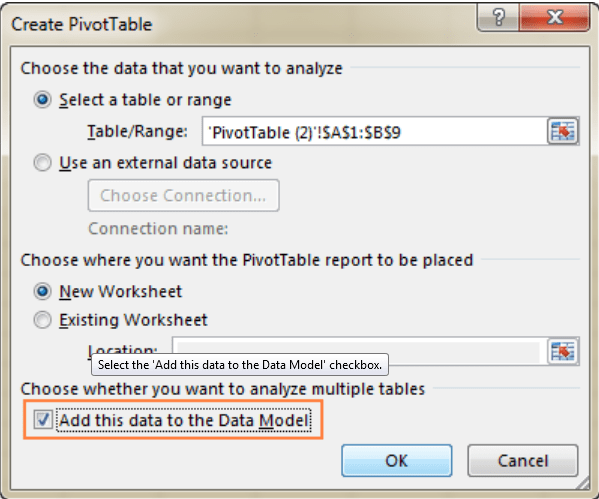• Move the field to Values area whose distinct values need to be counted. Click on the drop-down menu, and select Field Value Settings from the list.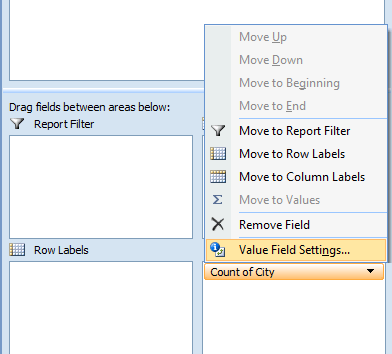• The Value Field Settings dialog opens. Scroll down to the Distinct Count, and press OK button.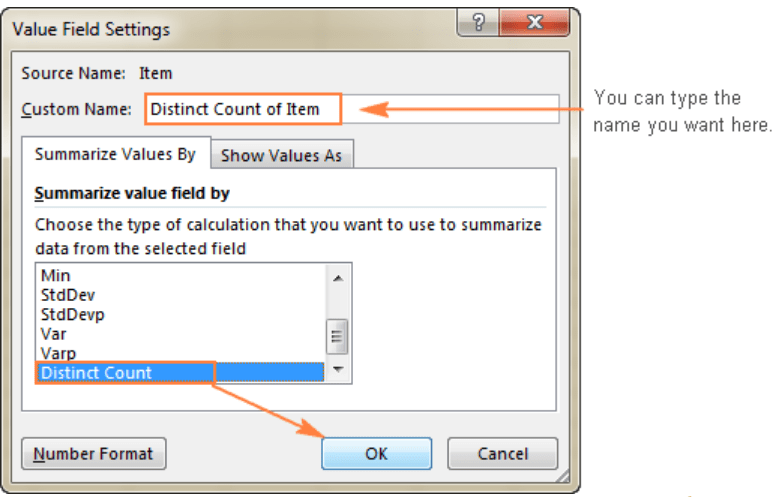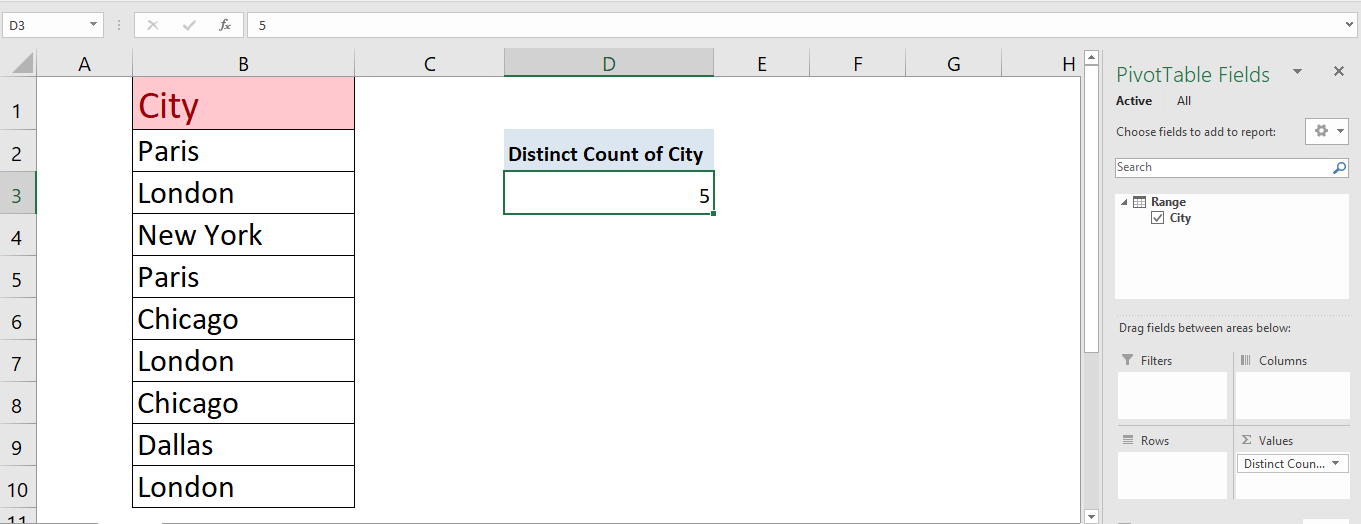The problem you are trying to solve could be more complex, if you are in a rush, or need a real Expert to help you save hours of struggle, click on this link to enter your problem and get connected to a qualified Excel expert in a few seconds. You can share your file, and an expert will create a solution for you on the spot during a 1:1 live chat session. The first session is free.

### Did this post not answer your question? Get a solution from connecting with the expert.Another blog reader asked this question today on Excelchat:
Related blogs
Solution examplesI am trying to have a tab change color when the Sum of a row = 0. I am using code on the tab but it appears to be confused because the value of the given cell is not 0 it is "=SUM(G4:G17)"
Solved by S. F. in 41 minsCould you help me by explaining the IF function?
Solved by G. L. in 24 minsI need a formula to compare the data in two columns and then export the mismatched data in the 3rd column
Solved by S. Q. in 20 minsI have a question about Conditional Formatting. =IF('Sheet1'!A1="X",IF('Sheet1'!B1="Y", TRUE, FALSE),FALSE) the set the cell background to red. I would like to make it so that if Sheet1'!B1="Z" I set the back to blue. How can I achieve this.
Solved by B. F. in 39 minsI need to find out if the 2018 yearly sales goals were met if the yearly sales were \$25,000 or more using an IF logical function and to set the formula to return a value of YES if met, and NO if not
Solved by A. A. in 18 mins## Subscribe to Excelchat.coAnother blog reader asked this question today on Excelchat: